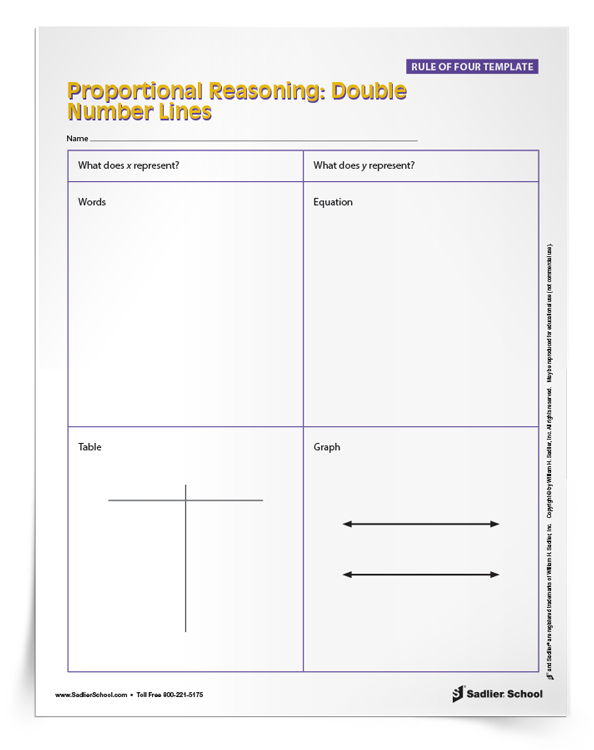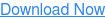1.800.221.5175
Core Program
Full Access
Core Program
Full Access
High-Impact Tutoring
Core Program
Full Access
National Program
Full Access
New York
Critical Thinking for Active Math Minds
Preparing for Standards Based Assessments
Vocabulary Workshop, Tools for Comprehension Grades 1–5
Print Program
Interactive Edition
Print Program
Interactive Edition
Vocabulary Workshop, Tools for Excellence Grades 6–12+
Print Program
Interactive Edition
Vocabulary for Success
Progress English Language Arts Grades K–8
National Program
Full Access
High-Impact Tutoring
New York
Grammar Workshop, Tools for Writing
Grammar for Writing
Print Program
Interactive Practice Bundle
Fluency Booster Practice Book
Interactive Assessments
High-Impact Tutoring
Building Reading Success with Wiley Blevins
Print Program
Interactive Edition
Professional Learning

A K–8 resource to support deep comprehension of math skills and conceptsTopics:

#### November 22, 2019 6-8-math-practices, mp-modeling

This post looks at a Rule of Four Template and how teachers can use it to help students model with mathematics at the middle school level. Plus, download four FREE printable Rule of Four Templates that can be used in grades 6–8 classrooms!

# Students Are Required to Model With Mathematics

Mathematical Practice 4 requires students to apply mathematics in order to solve problems. One example of this standard is having students model a situation by writing an equation or inequality. Students need to understand the mathematical situation presented and translate it into an equation or inequality.

On newer types of standardized tests, students are required to model with mathematics. An example of this kind of test is the PARCC math test, in which 18% of the test’s raw score points come from three open-response questions that require modeling.

# What is the Rule of Four?

The Rule of Four in mathematics education was developed as a guiding principle by the Harvard Calculus Consortium. It was conceived at Harvard University as part of their math reform effort, which was designed to deepen students’ conceptual understanding. The Rule of Four stipulates that topics in mathematics should be presented in four ways: geometrically, numerically, analytically, and verbally. Implementing the Rule of Four supports students in being adept with all four types of representations and also provides support to students who learn in different ways.

## How to Use the Rule of Four Template

The Rule of Four Template was developed by my state department of education as a part of a model curriculum unit. The Rule of Four Template requires students to represent every problem in four ways to model with mathematics:

1. Verbally (or in writing)

2. Algebraically (as an equation or inequality)

3. Geometrically (as a graph)

4. Numerically (in a table)

I have been using the Rule of Four Template to help my students build their understanding linear equation word problems by applying Mathematical Practice 4. When I teach students how to write linear functions in standard or slope-intercept form from a word problem, I find the Rule of Four Template helpful because students understand that various forms or models of the linear relationship represent the same thing.

As teachers, it is natural for us to understand that the points on a line are the solutions to linear equations, that those points can be listed in an organized table, and that unit rates and intercepts of the graph can be found in the word problem, but that isn’t always the case for our students.

Using the Rule of Four Template builds students’ capacity to use Mathematical Practice 4 and bring this understanding to light.

## Printable Rule of Four TemplatesWhile there are many mathematical topics where the Rule of Four Template can be used to model with mathematics, this post’s download focuses on three areas that you can use in middle school.

The first Rule of Four Template is for double number line ratio and proportion problems for Grade 6.

The second Rule of Four Template is for solving problems using inequalities for Grades 6 and 7. In sixth and seventh grade, I am providing a template with a number line diagram for inequality problems.

The third and fourth Rule of Four Templates are for seventh and eighth grade linear relationship problems, one for problems with a first quadrant graph, and the other for a graph with all four quadrants.